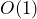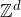# Gourab Ray and Yinon Spinka: Graphical representations and gradients of Ising as factors of i.i.d.

Abstract: A process on a graph is a factor of i.i.d. if it can be represented as an automorphism-equivariant function of i.i.d. random variables attached to its vertices, or in other words, there is a “local algorithm” which takes in i.i.d. random variables on the vertices as input, and outputs a sample of the process. Furthermore if for any vertex the “local algorithm” only needs to read the i.i.d. variables from a finite (but random) neighborhood of that vertex, we call the factor finitary.

Previous works showed that the plus/minus state of the Ising model on the Euclidean lattice or any transitive amenable graph is always a factor of i.i.d. and is a finitary factor of i.i.d. if and only if the temperature is at or above criticality. Such questions are not fully understood in the nonamenable setting, even on a regular tree. An important tool in all this analysis is that the Ising model is monotone.

We prove
— The loopmodel (a non-monotone graphical expansion of Ising) is a factor of i.i.d. in various settings (joint with Omer Angel). As a corollary: the gradient of the free state of Ising on planar graphs (even nonamenable) is a factor of i.i.d. This answers a question of Hutchcroft.
— The gradient of the plus/minus/free state of Ising (which is also non-monotone) is a finitary factor of i.i.d. at all temperatures in.

We will review some of the standard techniques for proving such results in monotone models and then explain how we build on them in these cases to prove our results.

## Video: